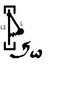# Pendulum & Spring Equation of Motion

• mathfan
In summary, the student is attempting to solve for the equation of motion for a system with a spring and dissipation. The student has to account for the elasticity and damping of the spring, as well as the angular velocity of the pendulum.

## Homework Statement

I have to derive equation of motion for this system. I want to use a moment of force, but i have a problem with moment of force spring.

## The Attempt at a Solution

What I've done is:
M(Fg)=-mgLsinα
M(N)=0
M(Fb)=mω^2 Lsinα*Lcosα
mL^2*α''=ΣM
M(Fs)=?

#### Attachments

•Beztytułu.jpg
2.8 KB · Views: 407
You still haven't provided the full problem statement.
Nor any equations.
Are you supposed to do this classically, or with a Lagrangian ?
And, as I stated, ##F= - kx## is usual for a spring.

Did you read through the guidelines ?

So, i have to find equation of motion for this system. We know length of the line (L) and L1(picture). Initially the angle between spring and line(L) is 90, so initial length of the spring is √(L1^2-L^2). What's more the the pendulum rotates around the main rod (
angular velocity ω).
Yes my post was deleted.

That all you have to describe the system (the full problem statement) ?
If so, are you allowed to make a small-angle approximation (##x = x_0 + L(\theta-\theta_0)## ) ?
If not, you have some trig to work out.
BvU said:
Are you supposed to do this classically, or with a Lagrangian ?

I'm supposed to this classically, but I'm not allowed to make a small-angle approximation. There is also dissipation F=-cl'. I can't even imagine how this system works. I worked out length of the spring (dependent on angle) using law of cosines, but i don't know if it's a good idea.

Last edited:
Pretty awkward indeed. Don't know how to make it simpler, I'm afraid ...Apparently the full problem statement is still more involved ? Although -cL' is a constant (?)

No -cl' isn't constatnt, l(small L) is the length of the spring(dependent on the angle)

That's not dissipation ! That's the spring (what I called ##
F= - kx ## ). Very nifty to use l' and L' for different quantities. Confusion assured !

The whole thing looks a bit like a steam engine governor with an extra spring: ##\omega## pushes the weight outward, gravity + spring pull it back.

mathfan said:
initial length of the spring is √(L1^2-L^2).

And if it doesn't say what the equilibrium length is, you're stuck ! (unless your problem statement says somehow that it is zero).
BvU said:
You still haven't provided the full problem statement

In my exercise is:
Assume forces:
1. Spring elasticity: F=-kΔl
2. Dissipation/damping: F=-cl'
So I don't think it's the same.

So l' is rate of change of l?

I still don't see a complete statement of the problem.

Yes l' is a derivative. It is all I have in my assignment